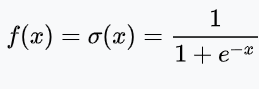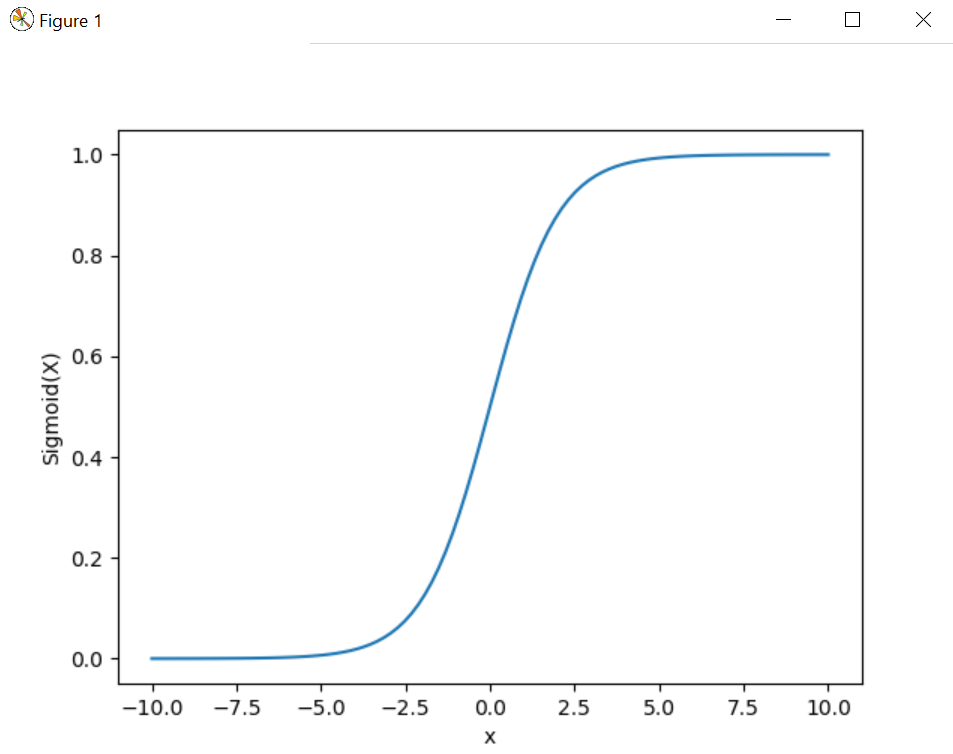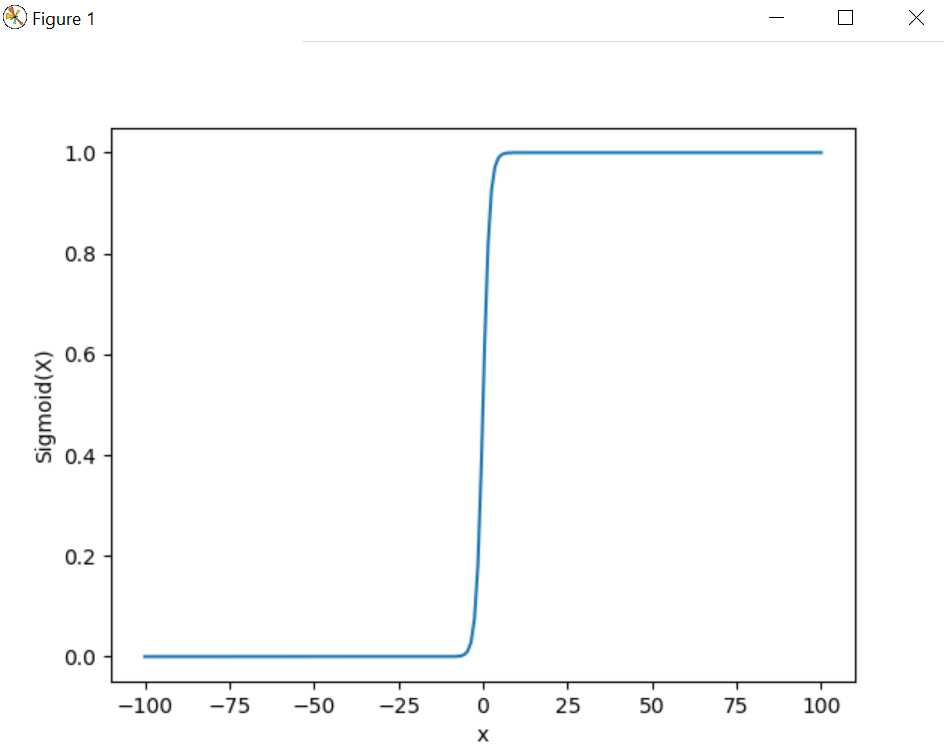# Implement sigmoid function using Numpy

• Last Updated : 03 Oct, 2019

With the help of Sigmoid activation function, we are able to reduce the loss during the time of training because it eliminates the gradient problem in machine learning model while training.`# Import matplotlib, numpy and math``import` `matplotlib.pyplot as plt``import` `numpy as np``import` `math`` ` `x ``=` `np.linspace(``-``10``, ``10``, ``100``)``z ``=` `1``/``(``1` `+` `np.exp(``-``x))`` ` `plt.plot(x, z)``plt.xlabel(``"x"``)``plt.ylabel(``"Sigmoid(X)"``)`` ` `plt.show()`

Output :Example #1 :

 `# Import matplotlib, numpy and math``import` `matplotlib.pyplot as plt``import` `numpy as np``import` `math`` ` `x ``=` `np.linspace(``-``100``, ``100``, ``200``)``z ``=` `1``/``(``1` `+` `np.exp(``-``x))`` ` `plt.plot(x, z)``plt.xlabel(``"x"``)``plt.ylabel(``"Sigmoid(X)"``)`` ` `plt.show()`

Output :My Personal Notes arrow_drop_up